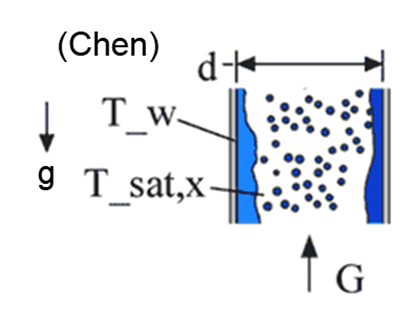Contents - Index

Flow_Boiling_ChenProcedure Flow_Boiling_Chen(Fluid\$, T_sat, G, d, x, T_w: h, q``) implements the Chen (1966) correlation for boiling heat transfer with saturated fluids in convective flow to determine the heat transfer coefficient for a boiling fluid in a vertical tube considering both convective and nucleate boiling contributions.

Inputs

Fluid\$ - string variable representing a real fluid in the EES data base.

T_sat - the saturation temperature of the incoming vapor in  [C], [K], [F] or [R] (units depend on unit system selected in EES).

G - mass velocity, i.e., the ratio of the mass flow rate to the cross-sectional area of the tube [kg/s-m^2] or [lbm/hr-ft^2]

d - diameter of the tube [m] or [ft].

x - quality (must be between 0 and 1)

T_w - wall temperature [C], [K], [F], or [R]

Outputs

h - heat transfer coefficient [W/m^2-K] or [Btu/hr-ft^2-F] including convective and nucleate boiling contributions.

q``- surface heat flux [W/m^2] or [Btu/hr-ft^2]

Notes:

1.  This procedure is responsible for determining the property data of the specified fluid . Fluid\$ must be a provided with a string variable or string constant that is one of the (non-ideal gas) fluids in EES.

Example:

\$UnitSystem SI Mass J K Pa

F\$='R245fa'

T_sat=300 [K]                                   "boiling saturation temperature"

G=200 [kg/m^2-s]

d=0.01 [m]

x=0.9                                                 "quality"

T_w=303 [K]

Call flow_boiling_chen(F\$, T_sat, G, d, x, T_w: h, q``)

{Solution: h=4523 [W/m^2-K]  and q``=13,568 [W/m^2]}